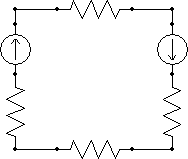QuickField

A new approach to field modelling
 Language: Global English Deutch Espanol Francais Italiano Danmark Ceske Chinese Pycckuü

Main >> Support >> Glossary >> Magnetic circuit reluctance

# Magnetic circuit reluctance*

The magnetic system's core can be represented as a magnetic circuit carrying the magnetic flux. The magnetic circuit reluctance can be estimated using the simulation software.Magnetic circuit reluctance may be calculated as Rm = Um / Flux,
where Rm - magnetic circuit resistance
Um = Integral(HdL) - magnetic voltage drop,
Flux - magnetic flux across the cross-section.

In case of uniform magnetic flux distribution the magnetic circuit reluctance can be calculated as

Rm = (1/μμ0) · L / SThe magnetic circuit analysis can be performed by the same methods as the electric circuit analysis. This approach is suitable for simple geometry and uniform flux distribution.

For complex core construction and non-linear materials the FEA approach is more appropriate. Depending on the problem formulation, you can use DC, AC or Transient Magnetics module of QuickField to calculate the magnetic circuit reluctance of the core.

• More about magnetic circuit reluctance simulation:
Magnetic field simulation in the air gap of DC electric motor.

• Magnetic circuit reluctance in the recorded webinar:
QuickField analysis for Electromagnetic Plunger design.